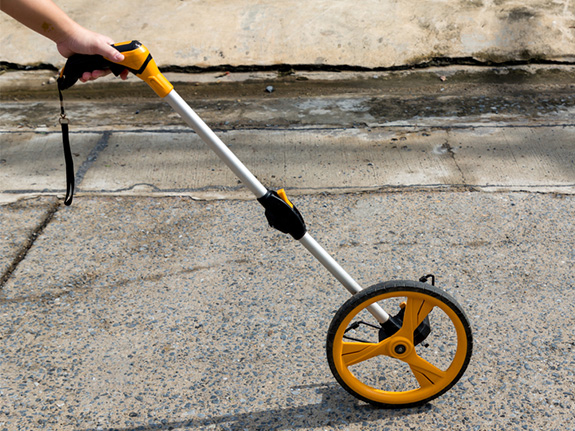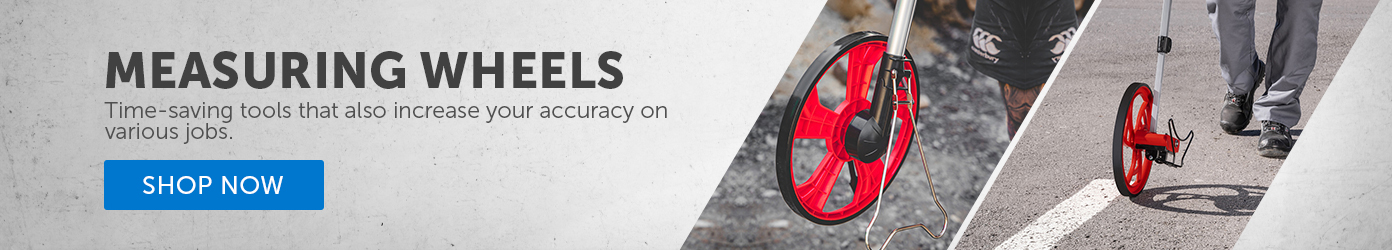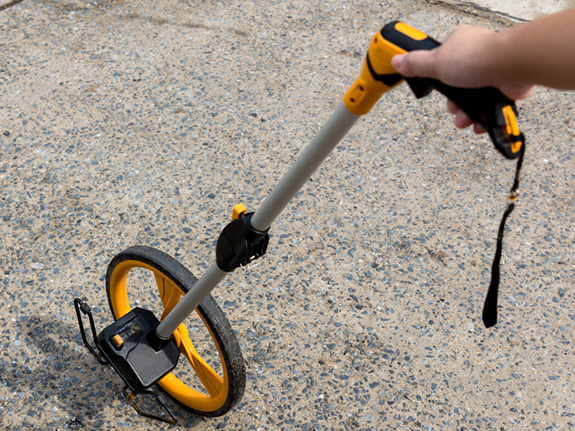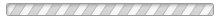Find Square Footage With a Measuring Wheel | Engineer Supply - EngineerSupply

# How to determine square footage using a measuring wheel?## How To Use a Measuring Wheel To Determine Square Footage

When measuring interior or exterior square footage, you can use a measuring wheel to take relatively accurate measurements. Find out how measuring wheels work, along with formulas for calculating the square footage of areas of different shapes and conversions from square feet to other common units of measurement. Learn more about the pros and cons of measuring wheels in terms of accuracy, affordability and ease of use.

## How Measuring Wheels WorkMeasuring wheels roll over the ground and keep track of the distance covered. Measuring wheels are available in diameters that range from 4 inches up to over 15 inches. Select a wheel diameter based on the distance and type of terrain you plan to cover with a wheel measuring tool.

Smaller measuring wheels are suitable for taking measurements indoors, while larger wheels are better suited for outdoor applications. Wheels that have diameters of 6 inches or larger are better for taking measurements over dirt, grass or other rough terrain. In addition to the wheel itself, many measuring wheels also have digital features such as measurement memory or calculation functions.

## How To Determine Square Footage

Calculate square footage by using an area formula for the right shape. While some smart measuring wheels can perform these calculations, a reminder of the formulas for calculating the area of different shapes and converting square footage calculations into different units of measurement can be beneficial.

Different formulas are used to calculate the area of rectangle, square, circle, triangle or trapezoid shapes. Here are a few equations that are helpful for calculating square footage:
• Rectangle: length x width
• Square: side length x side length
• Triangle: 1/2 (base × height)
• Trapezoid: ((a + b) / 2 )h
To determine total square footage, all you need to do is add the square footage of all areas together. Convert a measurement in square feet to square inches by multiplying the first value by 144, or convert square feet into square meters by multiplying the first value by 0.092903.

## Measuring Wheel Pros and Cons

Measuring wheels are often more affordable than laser measuring tools. A wheel may also take less time than an LDM to set up and calibrate prior to use. It is worth noting that even the most advanced wheels for measurement are considerably less accurate than laser systems that are rated as being accurate to ⅛ of an inch, let alone advanced precision measurement systems that are rated for accuracy to 1/16 of an inch.## Where can I buy a measurement wheel?Browse a large inventory of measuring wheels for sale at Engineer Supply. Choose from small, medium or large wheel sizes. You can also find digital wheels that have smart features such as memory for measurements and calculation functions. The right wheel can simplify square footage calculations based on accurate measurements.

## How do I calculate square footage?

Calculate the square footage of a rectangular area by multiplying the length measurement in feet by the width in feet. For a square-shaped area, multiply the length of one side by the length of another side. Other formulas are used to calculate the area of a circle, triangle or trapezoid.

## How do I take more accurate measurements with a wheel?

Wheels measure by rolling. Make sure a wheel is zeroed out before starting to measure and clear a path in a straight line between the measurement start and end points. Maneuvering a wheel around obstacles can lead to inaccurate measurements, and wheels are already less accurate than laser distance meters.

## What are some features of digital measuring wheels?

Digital measuring meters may be able to recall a set amount of past measurements and use these values in calculations such as area or square footage. A Bluetooth-enabled wheel or a measuring device with another type of wireless connectivity makes it easy to record measurements on a computer or device.

## Are laser distance meters more accurate than measuring wheels?

Laser distance meters are accurate to ⅛ or even 1/16 of an inch, while measuring wheels are only accurate to within 3 inches per 100 feet. You must properly calibrate an LDM and place targets or roll a wheel as directed to obtain accurate measurements for use in calculations.

When you use a measuring wheel to determine the length of each side of the perimeter or diameter of an area, you can obtain relatively accurate values for use in calculating the square footage of any area. Shop the full selection of measuring wheels available at Engineer Supply to select the best measuring tool for any project.
Please Wait...